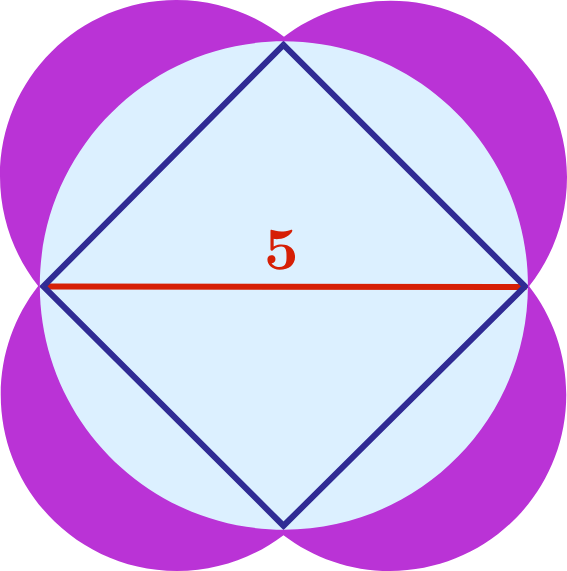# 50 of 100: Lumière des Lunes

Geometry Level 2The four sides of a square are used as diameters to define four overlapping, congruent circles. Then, a larger circle is drawn so that it passes through all four corners of the square. The length of the square's diagonal is 5.

What is the total area of the regions shaded purple?Hint: In one approach to solving this puzzle, you start by finding the length of one side of the square.

×